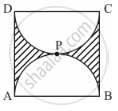Share

# Find the Perimeter of the Shaded Region in Figure 4, If Abcd is a Square of Side 14 Cm and Apb and Cpd Are Semicircles. Use π 22 7 - CBSE Class 10 - Mathematics

ConceptAreas of Combinations of Plane Figures

#### Question

Find the perimeter of the shaded region in Figure 4, if ABCD is a square of side 14 cm and APB and CPD are semicircles. Use pi22/7#### Solution

The length of the sides of square ABCD is 14 cm.

∴ AB = BC = CD = AD = 14 cm

Radius of each semi-circular portion 14/2 cm =7 cm

Perimeter of the shaded region = Perimeter of semi-circle APB + perimeter of semi-circle CPD + BC + AD

\text{Perimeterof semi - circle APB}=1/2xx2pixxradius=1/2xx2pixx7 cm =22/7 xx7cm =22cm

\text{Perimeterof semi - circle CPD}=1/2xx2pixxradius=1/2xx2pixx7 cm =22/7 xx7cm =22cm

∴ Perimeter of the shaded region = 22 cm + 22 cm + 14 cm + 14 cm = 72 cm

Is there an error in this question or solution?

#### APPEARS IN

Solution Find the Perimeter of the Shaded Region in Figure 4, If Abcd is a Square of Side 14 Cm and Apb and Cpd Are Semicircles. Use π 22 7 Concept: Areas of Combinations of Plane Figures.
S